Courses

# RD Sharma Solutions (Part - 1) - Ex-14.2, Lines and Angles, Class 7, Math Class 7 Notes | EduRev

## Class 7 : RD Sharma Solutions (Part - 1) - Ex-14.2, Lines and Angles, Class 7, Math Class 7 Notes | EduRev

The document RD Sharma Solutions (Part - 1) - Ex-14.2, Lines and Angles, Class 7, Math Class 7 Notes | EduRev is a part of the Class 7 Course RD Sharma Solutions for Class 7 Mathematics.
All you need of Class 7 at this link: Class 7

#### Question 1:

In Fig., line n is a transversal to lines l and m. Identify the following: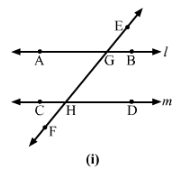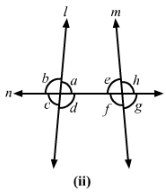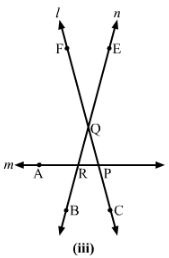(i) Alternate and corresponding angles in Fig. (i).
(ii) Angles alternate to ∠d and ∠g and angles corresponding to angles ∠f and ∠h in Fig. (ii).
(iii) Angle alternate to ∠PQR, angle corresponding to ∠RQF and angle alternate to ∠PQE in Fig. (iii).
(iv) Pairs of interior and exterior angles on the same side of the transversal in Fig. (ii).

(i) Figure (i)
Corresponding angles:
∠EGB and ∠GHD
∠HGB and ∠FHD
∠EGA and ∠GHC
∠AGH and ∠CHF
Alternate angles:
∠EGB and ∠CHF
∠HGB and ∠CHG
∠EGA and ∠FHD
∠AGH and ∠GHD

(ii) Figure (ii)
Alternate angle to ∠d is ∠e.
Alternate angle to ∠g is ∠b.
Also,
Corresponding angle to ∠f is ∠c.
Corresponding angle to ∠h is ∠a.

(iii) Figure (iii)
Angle alternate to PQR is QRA.
Angle corresponding to RQF is ARB.
Angle alternate to POE is ARB.

(iv) Figure (ii)
Pair of interior angles are
a and ∠e
d and ∠f
Pair of exterior angles are
b and ∠h
c and ∠g

#### Question 2:

In Fig., AB and CD are parallel lines intersected by a transversal PQ at L and M respectively. If ∠CMQ = 60°, find all other angles in the figure.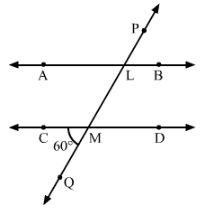∠ALM = ∠CMQ = 60°60°        (Corresponding angles)
∠LMD = ∠CMQ = 60°60°        (Vertically opposite angles)
∠ALM = ∠PLB = 60°60°          (Vertically opposite angles)
Since
∠CMQ + ∠QMD = 180°180°     (Linear pair)
∴ ∠QMD = 180°−60°=120°
∠QMD = ∠MLB = 120°        (Corresponding angles)
∠QMD = ∠CML = 120°        (Vertically opposite angles)
∠MLB = ∠ALP = 120°          (Vertically opposite angles)

#### Question 3:

In Fig., AB and CD are parallel lines intersected by a transversal PQ at L and M respectively. If ∠LMD = 35° find ∠ALM and ∠PLA.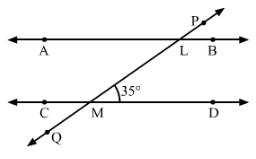In the given Fig., AB || CD.
∠ALM=∠LMD=35°     (Alternate interior angles)
Since ∠PLA+∠ALM=180°     (Linear pair)
∴∠PLA=180°−35°=145°

Question 4:

The line n is transversal to line l and m in Fig. Identify the angle alternate to ∠13, angle corresponding to ∠15, and angle alternate to ∠15.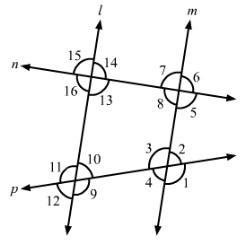In this given Fig., line l || m.
Here,
Alternate angle to ∠13 is ∠7.
Corresponding angle to ∠15 is ∠7.
Alternate angle to ∠15 is ∠5.

#### Question 5:

In Fig., line l || m and n is a transversal. If ∠1 = 40°, find all the angles and check that all corresponding angles and alternate angles are equal.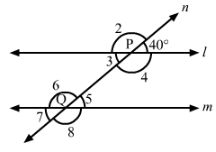In the given figure, l || m.
Here,
∠1+∠2=180°     (Linear pair)
∴ ∠2=180°−∠1=180°- 40°=140°
∠5=∠1=40°        (Corresponding angles)
∠3=∠1=40°        (Vertically opposite angles)
∠7=∠3=40°        (Corresponding angles)
∠7=∠5=40°        (Vertically opposite angles)

Also,
∠2=∠6=140°        (Corresponding angles)
∠2=∠4=140°        (Vertically opposite angles)
∠4=∠8=140°        (Corresponding angles)
∠8=∠6=40°          (Vertically opposite angles)

Thus,
∠2=∠8, ∠3=∠5, ∠6=∠4, ∠1=∠7
Hence, alternate angles are equal.

#### Question 6:

In Fig., line l || m and a transversal n cuts them at P and Q respectively. If ∠1 = 75°, find all other angles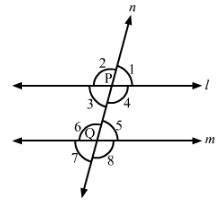In the given figure, l || m, n is a transversal line and ∠1 = 75°.
Thus, we have:
∠1+∠2=180°        (Linear pair)
⇒∠2=180°−∠1=180°−75°=105°
∴∠1=∠5=75°         (Corresponding angles)
∠1=∠3=75°             (Vertically opposite angles)
∠5=∠7=75°             (Vertically opposite angles)
Now,
∠2=∠6=105°         (Corresponding angles)
∠6=∠8=105°          (Vertically opposite angles)
∠2=∠4=105°          (Vertically opposite angles)

#### Question 7:

In Fig., AB || CD and a transversal PQ cuts them at L and M respectively. If ∠QMD = 100°, find all other angles.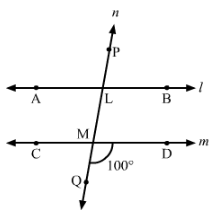In the given figure, AB || CD, PQ is a transversal line and QMD = 100°.
Thus, we have:
DMQ + QMC = 180°    (Linear pair)
∴∠QMC=180°−∠DMQ=180°−100°=80°

Thus,
∠DMQ = ∠BLM = 100°         (Corresponding angles)
∠DMQ = ∠CML = 100°         (Vertically opposite angles)
∠BLM = ∠PLA = 100°           (Vertically opposite angles)
Also,
∠CMQ = ∠ALM = 80°         (Corresponding angles)
∠CMQ = ∠DML = 80°         (Vertically opposite angles)
∠ALM = ∠PLB = 80°           (Vertically opposite angles)

#### Question 8:

In Fig., l || m and p || q. Find the values of xyzt.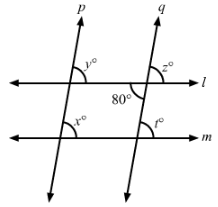In the given figure, l || m and p || q.
Thus, we have:
∠z=80°∠z=80°                (Vertically opposite angles)
∠z=∠t=80°∠z=∠t=80°       (Corresponding angles)
∠z=∠y=80°∠z=∠y=80°       (Corresponding angles)
∠x=∠y=80°∠x=∠y=80°       (Corresponding angles)

#### Question 9:

In Fig., line l || m, ∠1 = 120° and ∠2 = 100°, find out ∠3 and ∠4.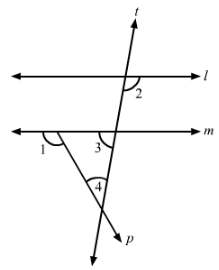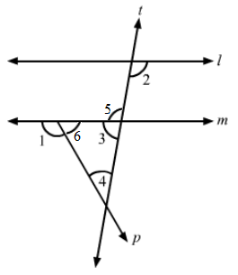In the given figure, ∠1 = 120° and ∠2 =100°.
Since l || m, so
∠2=∠5=100°              (Alternate interior angles)
∠5+∠3=180°                (Linear pair)
⇒∠3=180°−∠5=180°−100°=80°
Also,
∠1+∠6=180°          (Linear pair)
⇒∠6=180°−∠1=180°−120°=60°

We know that the sum of all the angles of triangle is 180°.
∴∠6+∠3+∠4=180°
⇒60°+80°+∠4=180°
⇒140°+∠4=180°
⇒∠4=180°−140°=40°

#### Question 10:

In Fig., line l || m. Find the values of abcd. Give reasons.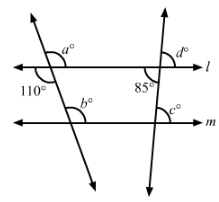In the given figure, line l || m.
Thus, we have:
∠a=110°        (Vertically opposite angles)
∠b=∠a=110°               (Corresponding angles)
∠d=85°           (Vertically opposite angles)
∠c=∠d=85°                  (Corresponding angles)

#### Question 11:

In Fig., AB || CD and ∠1 and ∠2 are in the ratio 3 : 2. Determine all angles from 1 to 8.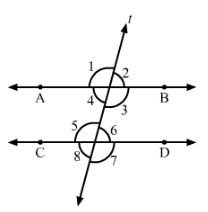In the given figure, AB || CD and t is a transversal line.
Now, let:
∠1=3x
∠2=2x
Thus, we have:
∠1+∠2=180°      (Linear pair)
∴ 3x+2x=180°
⇒5x=180°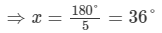Thus,∠1=3×36°=108°
∠2=2×36°=72°

Now,
∠1=∠5=108°      (Corresponding angles)
∠1=∠3=108°      (Vertically opposite angles)
∠5=∠7=108°      (Vertically opposite angles)
∠2=∠6=72°        (Corresponding angles)
∠4=∠2=72°        (Vertically opposite angles)
∠8=∠6=72°        (Vertically opposite angles)

#### Question 12:

In Fig., lm and n are parallel lines intersected by transversal p at XY and Z respectively. Find ∠1, ∠2 and ∠3.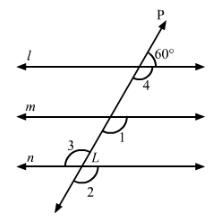In the given figure, l || m || n and p is a transversal line.
Thus, we have:
∠4+60°=180°       (Linear pair)
⇒∠4=180°−60°=120°∠4=∠1=120°       (Corresponding angles)
∠1=∠2=120°        (Corresponding angles)
∠3=∠2=120°         (Vertically opposite angles)
Thus,
∠1=∠2=∠3=120°

Offer running on EduRev: Apply code STAYHOME200 to get INR 200 off on our premium plan EduRev Infinity!

97 docs

,

,

,

,

,

,

,

,

,

,

,

,

,

,

,

,

,

,

,

,

,

,

,

,

,

,

,

,

,

,

;